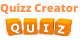Create Quiz

# Do You Know Angular Momentum?## This test is simple, straightforward, and standard. It measures takers knowledge about angular momentum which is a quantity of rotation of an object a

You can mute/unmute sounds from here

Path
Direction
Quantity
Distribution

## Angular momentum belongs to which of she's groups?

Derived quantities
Fundamental quantities
Scalar quantities
Force

Newton's law
Kepler 's law
Hooke's law
Pascal's law

L = IW
F = Ke
A = MK
W = 1/2 Ke

Axis
Level
Point
Path

Translational
Rectilinear
Harmonic motion
Rotational

M
L
A
K

Acceleration
Angular velocity
Force
Torque

Vector
Scalar
Linear
Rotational

## The total angular momentum of an object remains constant unless acted upon by an external

Force
Acceleration
Velocity
Torque

Currently, we have no comments. Be first to comment on this quiz.

SIMILAR QUIZZESGerontology – study of ageing processes Quiz
Gerontology – study of ageing processes quiz Test, this is for funProtein Synthesis biology Quiz
This quiz helps sharpen your prowess in the topic. It also offers you a chance to see how well you know this topic. Try it out.Captain America the first Avenger
These quiz is about Captain America the first Avenger movieGenomics - The Study of Genomes | Quiz test
Genomics is the study of whole genomes of organisms, and incorporates elements from genetics. Genomics uses a combination of recombinant DNA, DNA sequencing methods, and bioinformatics to sequence,...

## Do You Know Angular Momentum? : Test Trivia

Ultimate impossible quiz game

Embed This Quiz
Copy the code below to embed this quiz